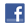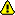Add a new lesson / test / 1 lesson per weekNew account
4 million accounts created!
JOIN our free club and learn English now!

• Home
• Print
• Guestbook
• Report a bug

•GREAT!
Get a free English lesson every week! Thousands of subscribers!

Partners:
- Our other sites

Learn English > English lessons and exercises > English test #325: Ordinal numbers> Other English exercises on the same topic: Numbers [Change theme] > Similar tests: - Fractions and percentages - ESL-Numbers from 1 to 10 - Geometric shapes - Vocabulary: mathematics - Idioms: numbers - Geometry-Vocabulary - Dozen(s), hundred(s), thousand(s), million(s)? - Numbers and dates... (Level 1) > Double-click on words you don't understand

Ordinal numbers

Write down these ordinal numbers.Twitter Share
English exercise "Ordinal numbers" created by anonyme with The test builder.
Click here to see the current stats of this English testPlease log in to save your progress.

1. the 33rd = the

2. the 57th = the

3. the 15th = the

4. the 40th = the

5. the 31st = the

6. the 55th = the

7. the 77th = the

8. the 65th = the

9. the 44th = the

10. the 89th = the

11. the 53rd = the

12. the 12th = the

End of the free exercise to learn English: Ordinal numbers
A free English exercise to learn English.
Other English exercises on the same topic : Numbers | All our lessons and exercises